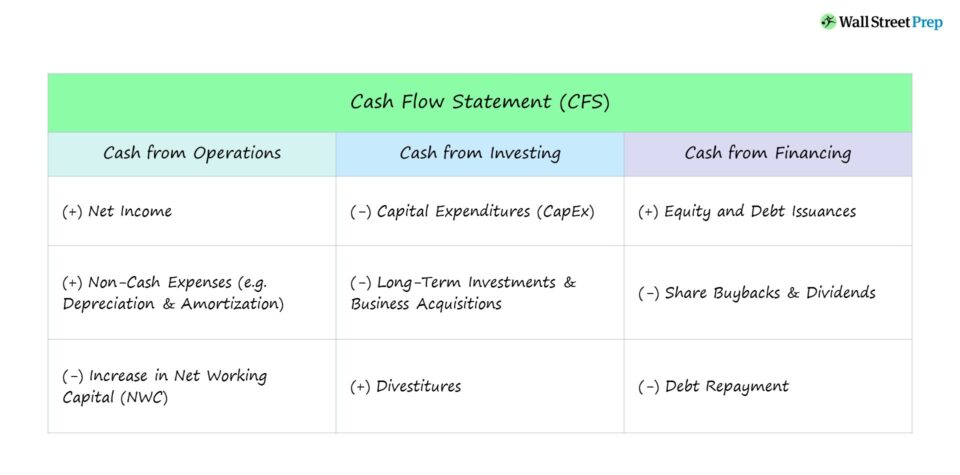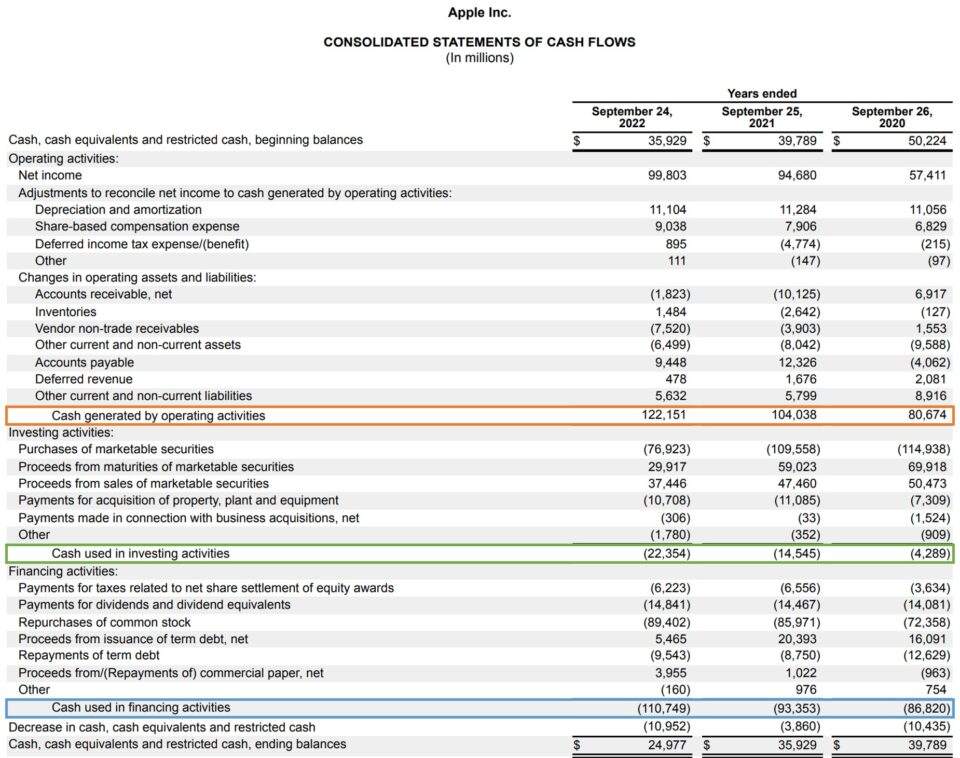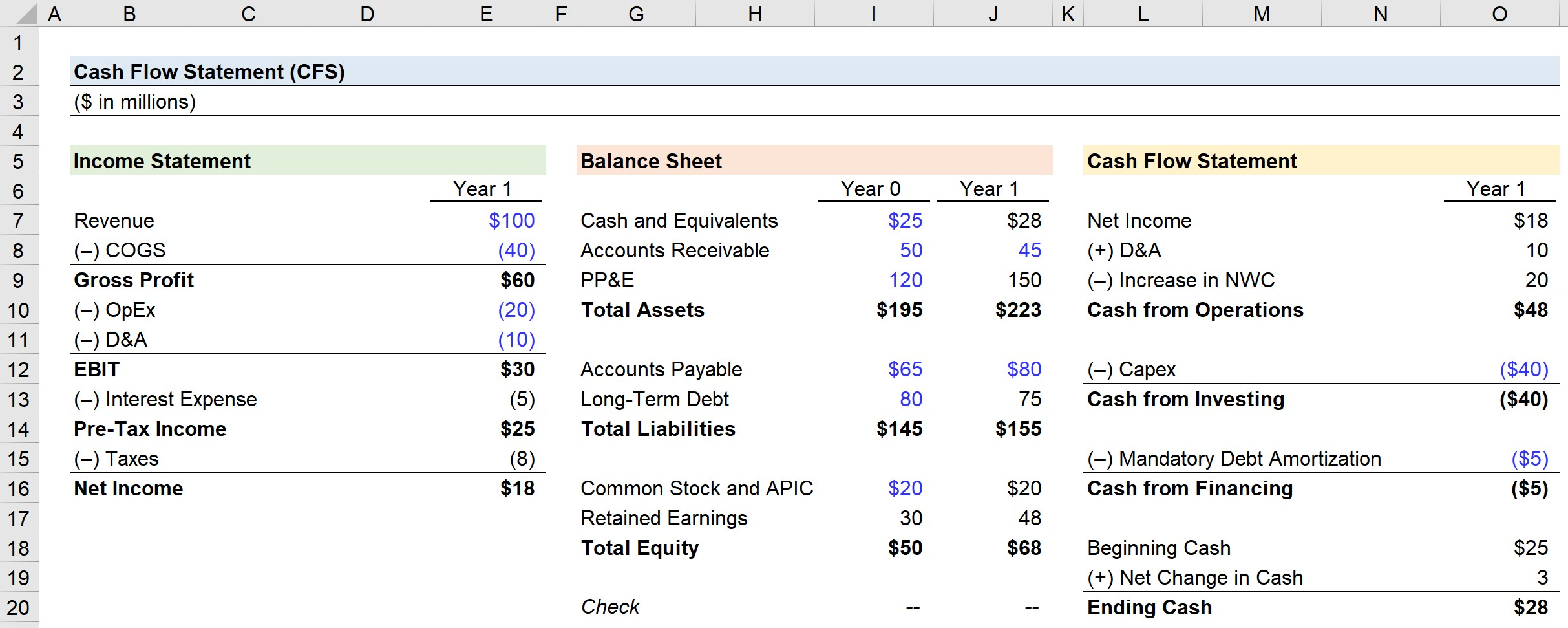Welcome to Wall Street Prep! Use code at checkout for 15% off.# Cash Flow Statement (CFS)

Guide to Understanding the Cash Flow Statement (CFS)## How to Understand Cash Flow Statement (Step-by-Step)

The statement of cash flows, or “cash flow statement”, along with the income statement and balance sheet, represent the three core financial statements.

The importance of the cash flow statement (CFS) is tied to the reporting standards established under accrual accounting.

The net income as shown on the income statement – i.e. the accrual-based “bottom line” – might not be an accurate depiction of what is actually occurring to the company’s cash.

Therefore, the statement of cash flows is necessary to reconcile net income to adjust for factors such as:

In effect, the real movement of cash during the period in question is captured on the statement of cash flows – which brings attention to operational weaknesses and investments/financing activities that do not appear on the accrual-based income statement.

The impact of non-cash add-backs is relatively straightforward, as these have a net positive impact on cash flows (e.g. tax savings).

However, for changes in net working capital, the following rules apply:

• Increase in NWC Asset and/or Decrease in NWC Liability ➝ Decrease in Cash Flow
• Increase in NWC Liability and/or Decrease in NWC Asset ➝ Increase in Cash Flow

Focusing on net income without looking at the real cash inflows and outflows can be misleading because accrual-basis profits are easier to manipulate than cash-basis profits. In fact, a company with consistent net profits could potentially even go bankrupt.

## Cash Flow Statement (CFS): Indirect Method vs. Direct Method

The two methods by which cash flow statement (CFS) can be presented are the 1) indirect method and 2) direct method.

Format Description
Indirect Method
• The indirect method is the standard format among U.S. companies, whereby the starting line item is net income.
• Net income is subsequently adjusted for non-cash items (e.g. depreciation & amortization) and changes in working capital to arrive at cash flow from operations.
Direct Method
• In the direct method, net income is not the starting point, but rather, the direct method explicitly lists the cash received and paid out to third parties during the period.
• For example, the flow of cash received from customers and the cash paid to suppliers.

## Statement of Cash Flows: Indirect Method Accounting Format

Under the indirect method, the cash flow statement is broken out into three distinct sections.

Format Description
1. Cash Flow from Operating Activities (CFO)
• The section’s top-line item is net income, which is adjusted by adding back non-cash expenses, such as D&A and stock-based compensation, and then adjusted for changes in working capital line items.
2. Cash Flow from Investing Activities (CFI)
• In the next section, investments are accounted for, with purchases of PP&E (i.e. capital expenditures, as the major recurring outflow), followed by business acquisitions and divestitures.
3. Cash Flow from Financing Activities (CFF)
• In the final section, the net cash impact of raising capital from issuing equity or debt from outside investors, share repurchases (i.e. buybacks), repayments of financial obligations, and the issuance of dividends are taken into account.

## Cash Flow Statement Formula (CFS)

If the three sections are added together, we arrive at the “Net Change in Cash” for the period.

Net Change in Cash = Cash from Operations + Cash from Investing + Cash from Financing

Subsequently, the net change in cash amount will then be added to the beginning-of-period cash balance to calculate the end-of-period cash balance.

Ending Cash Balance = Beginning Cash Balance + Net Change in Cash

The shortcomings regarding the income statement (and accrual accounting) are addressed here by the CFS, which identifies the cash inflows and outflows over a certain time span while utilizing cash accounting – i.e. tracking the cash coming in and out of the company’s operations.

## Cash Flow Statement Example: Apple (AAPL) Fiscal Year 2022

The following is a real world example of a cash flow statement prepared by Apple (AAPL) under GAAP accrual accounting standards.Apple Cash Flow Statement Example (Source: AAPL 10-K)

## How are the 3-Statements Linked?

Assuming the beginning and end of period balance sheets are available, the cash flow statement (CFS) could be put together (even if not explicitly provided) as long as the income statement is also available.

## Cash Flow Statement Calculator (CFS) – Excel Template

We’ll now move to a modeling exercise, which you can access by filling out the form below.Submitting...

## Step 1. How to Build Cash Flow Statement (CFS)

Suppose we are provided with the three financial statements of a company, including two years of financial data for the balance sheet.

The completed statement of cash flows, which we’ll work towards computing throughout our modeling exercise, can be found below.## Step 2. Income Statement Calculation (P&L)

In Year 1, the income statement consists of the following assumptions.

## Step 3. Cash Flow Statement Calculation (CFS)

The net income of \$18m is the starting line item of the CFS.

In the “Cash from Operations” section, the two adjustments are the:

• (+) D&A: \$10m
• (–) Increase in NWC: \$20m

Next, the only line item in the “Cash from Investing” section is capital expenditures, which in Year 1 is assumed to be:

• (–) Capex: \$40m

Likewise, the only “Cash from Financing” line item is the mandatory debt amortization (i.e. required pay down of debt principal):

• (–) Mandatory Debt Amortization: \$5m

The beginning cash balance, which we get from the Year 0 balance sheet, is equal to \$25m, and we add the net change in cash in Year 1 to calculate the ending cash balance.

• Cash from Operations: \$48m
• (+) Cash from Investing: -\$40m
• (+) Cash from Financing: -\$5m
• Net Change in Cash: \$3m

Upon adding the \$3m net change in cash to the beginning balance of \$25m, we calculate \$28m as the ending cash.

• Beginning Cash: \$25m
• (+) Net Change in Cash: \$3m
• Ending Cash: \$28m

## Step 4. Balance Sheet Calculation (B/S)

On the Year 1 balance sheet, the \$28m in ending cash that we just calculated on the CFS flows into the current period cash balance account.

For the working capital assets and liabilities, we assumed the YoY balances changed from:

• Accounts Receivable: \$50m to \$45m
• Accounts Payable: \$65m to \$80m

Operating assets declined by \$5m while operating liabilities increased by \$15m, so the net change in working capital is an increase of \$20m – which our CFS calculated and factored into the cash balance calculation.

For our long-term assets, PP&E was \$100m in Year 0, so the Year 1 value is calculated by adding Capex to the amount of the prior period PP&E and then subtracting depreciation.

• PP&E – Year 1: \$100m + \$40m – \$10m = \$110m

Next, our company’s long-term debt balance was assumed to be \$80m, which is decreased by the mandatory debt amortization of \$5m.

• Long-Term Debt – Year 1: \$80m – \$5m = \$75m

With the assets and liabilities side of the balance sheet complete, all that remains is the shareholders’ equity side.

The common stock and additional paid-in capital (APIC) line items are not impacted by anything on the CFS, so we just extend the Year 0 amount of \$20m to Year 1.

• Common Stock and APIC – Year 1: \$20m

The formula in Year 0 of the retained earnings balance serves as a “plug” for the accounting equation to remain true (i.e. assets = liabilities + equity).

But for Year 1, the retained earnings balance is equal to the prior year’s balance plus net income.

• Retained Earnings – Year 1: \$30m + \$18m = \$48m

Note that if there were any dividends issued to shareholders, the amount paid out would come out of retained earnings.

## Step 5. Financial Model Balance Check (Assets = Liabilities + Equity)

In our final step, we can confirm our model is built correctly by checking that both sides of our balance sheet in Year 0 and Year 1 are in balance.

• Accounting Equation: Assets = Liabilities + EquityStep-by-Step Online Course

### Everything You Need To Master Financial Modeling

Enroll in The Premium Package: Learn Financial Statement Modeling, DCF, M&A, LBO and Comps. The same training program used at top investment banks.Inline Feedbacksgerald
March 16, 2023 8:53 am

hello, I am wondering why taxes of \$8 were not deducted from the cash flow via the operating cashflows to get to \$40 from the \$48.March 16, 2023 3:44 pm

Hi, Gerald, The \$8 of tax expense is already included in the net income of \$18, which is the starting point of our CFO, so we do not need to subtract it again. However, non-cash items like D&A need to be added back to net income since they are non-cash… Read more »Learn Financial Modeling Online

Everything you need to master financial and valuation modeling: 3-Statement Modeling, DCF, Comps, M&A and LBO.

X

The Wall Street Prep Quicklesson Series

7 Free Financial Modeling Lessons

Get instant access to video lessons taught by experienced investment bankers. Learn financial statement modeling, DCF, M&A, LBO, Comps and Excel shortcuts.